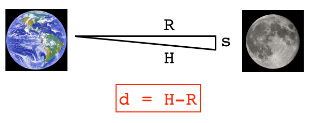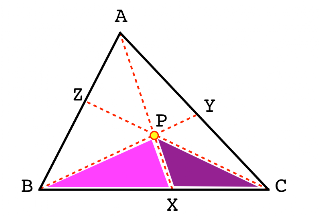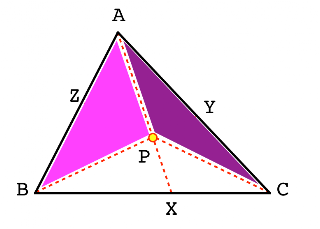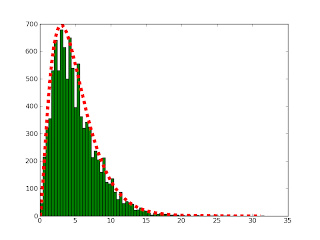## Tuesday, April 27, 2010

### Duly quoted

I have always imagined that paradise will be a kind of library.

--- Jorge Luis Borges

Sighted on AbeBooks---a favorite site. info on source

## Sunday, April 25, 2010

### Harmony of the sphere

I've been looking for a good derivation of the volume of a sphere, suitable for students of geometry. I found one in Precalculus Mathematics in a Nutshell by Simmons (Amazon). This focused little book is a perfect summary of pre-calculus math.

The method used is a little sneaky, as it seems to be a Riemann sum in disguise. But it doesn't really feel like calculus, and simply relies on two formulas for series, one for the sum of the integers between 1 and n, and the second for the sum of the squares of the integers:

 1 + 2 + .. n = n(n+1)/212 + 22 + .. n2 = n(n+1)(2n+1)/6

Consider a hemisphere with radius r, as shown in the figure.We'll divide it up into horizontal slices, figure the volume of each, and then add up the volumes for all of the slices, yielding the volume of the entire hemisphere. Although the volumes for the individual slices are not quite right (because x isn't the same at the top and bottom of the slice), if we make the slices thin enough, this won't matter.

Cut the sphere into n slices. To make the math simpler, we'll throw one away, considering only n-1 of them. If this bothers you, remember that eventually n will become very large, so that n is nearly equal to n-1.

The width of each slice is r/n. Let y = 0 at the top.

Enumerate the slices with the index k. For the kth slice, y = kr/n. And by the Pythagorean theorem:

 x2 = r2 - (r-y)2. = 2ry - y2 = 2r(kr/n) - (kr/n)2

The volume of each slice is then:

 width times arear/n πx2 = = π r/n [2r(kr/n) - (kr/n)2] = πr3 [2(1/n)2k - (1/n)3k2]

We need to add up these values for k = 1 to k = n - 1. Take the terms in the brackets one at a time. The first is:

 2 (1/n)2 (Σk)

Using the standard formula that the sum of the integers from 1 to n = n(n+1)/2, canceling the 2 and remembering that we're actually summing from 1 to n-1:

 (1/n)2 (n-1) n

Notice that we have just enough n's on the bottom to cancel. For the (n-1) term, dividing by n we get (1 - 1/n) ≈ 1. The entire first term reduces to:

  (1) (1-1/n) = 1

The second term is:

 -(1/n)3 (Σk2)

Using the standard formula that the sum of the squares of the integers from 1 to n = n(n+1)(2n+1)/6, and remembering that we're summing from 1 to n-1:

 -(1/n)3 (n-1)(n)(2n-1)/6

Again, we have just enough n's on the bottom to cancel.

 (1/n)3 (n-1)(n)(2n-1)/6 = = (1-1/n)(1)(2-1/n)/6 = 1/3

Putting it all together, we have

 πr3 (1 - 1/3) =2/3 πr3

The complete sphere is twice that:

 V = 4/3 πr3

That was painless!

## Tuesday, April 20, 2010

### Area under the curveI had a post the other day about the product rule in differential calculus, and how to extend it to find the derivative of a reciprocal and a quotient. The product rule also does heavy duty in the opposite direction, in the method called integration by parts. Strang has a wonderful diagram of that (shown above), which I've reproduced here using matplotlib.

The product rule is:

 (uv)' = u v' + v u'

Integrating both sides and isolating u v' we get:

 ∫u v' = u v - ∫v u'

where for the definite integral we have to remember to evaluate u times v at both of the limits of integration, subtracting the value at the lower limit from the upper one.

 ∫u v' = u2 v2 - u1 v1 - ∫v u'

Can you see each of these four terms as areas in the diagram? It's very pretty.

 from math import sqrtimport numpy as npimport matplotlib.pyplot as plt@np.vectorizedef f(x): return 100*sqrt(x) + 0.2*x**3# the curvedx = 0.01X = np.arange(0,10,dx)plt.plot(X,f(X),'r',lw=6,zorder=1)# the pointsu1 = 2u2 = 10plt.scatter(u1,f(u1),s=250, color='k',zorder=2)plt.scatter(u2,f(u2),s=250, color='k',zorder=2)# u dvX2 = np.arange(u1,u2+dx,dx)plt.fill_between(X2,f(X2),0, color='0.85',zorder=0)# v duYMAX = f(10)plt.fill_between(X2,YMAX,f(X2), color='0.35',zorder=0)X3 = np.arange(0,u1+dx,dx)plt.fill_between(X3,YMAX,f(u1), color='0.35',zorder=0) plt.text(6,100,s='$\int$ $u$ $dv$', fontsize=24)plt.text(2,300,s='$\int$ $v$ $du$', fontsize=24,color='w')plt.text(0,525, s='$y = 100 \sqrt{x}$ + $0.2 {x^3}$', fontsize=24,color='r')plt.text(2.5,115,s='$u_1$,$v_1$', fontsize=18)plt.text(10.5,500,s='$u_2$,$v_2$', fontsize=18)ax = plt.axes()r1 = plt.Rectangle((0,0),width=u1, height=f(u1),facecolor='cyan')ax.add_patch(r1)ax.set_xlim(-1,12.2)ax.set_ylim(-10,600)plt.savefig('example.png')

## Thursday, April 15, 2010

### Comparing apples and oranges?

I have a post about Newton's brilliant insight that gravity as observed on earth could be extended to the moon. You can read about this lots of places, I like James Gleick's Isaac Newton (Amazon).
Many years later Newton told at least four people that he had been inspired by an apple in his Woolsthorpe garden--perhaps an apple actually falling from a tree, perhaps not. He never wrote of an apple. He recalled only:
I began to think of gravity extending to the orb of the Moon . . .

---gravity as a force, then, with an extended field of influence; no cutoff or boundary---
& computed the force requisite to kep the Moon in her Orb with the force of gravity at the surface of the earth . . . & found them answer pretty nearly. All this was in the two plague years of 1665-1666. For in those days I was in the prime of my age for invention & minded mathematicks and Philosophy more than at any time since.

The apple was nothing in itself. It was half of a couple---the moon's impish twin. As an apple falls toward the earth, so does the moon; falling away from a straight line, falling around the earth.

---Gleick
Isaac Newton (p. 55)

The force acting on the moon or the apple is proportional to the mass for each, but the acceleration imparted is in turn proportional to that force divided by the mass, which cancels the mass term (for apple and moon).

This leaves an equation relating the acceleration to a constant (G) times the mass of the earth (M) divided by the square of the distance to earth. Thus, we expect that the acceleration (and the distance moved) for the moon and the apple to be in the inverse ratio as the square of their distance from the earth.

 a = GM/r2

The key relationship is shown in the diagram.We draw the tangent to the orbital circle at time-zero at a right angle with the radius. Then the distance moved in one second, s, is the velocity times the time. The hypotenuse, H, is calculated from R and s by the Pythoagorean Theorem. And the distance "fallen" in the direction of the earth is the difference between H and the radius, R.

 R = EM distanceH = sqrt (R2 + (radial velocity * 1 sec)2)d = H - R

d is very small compared to R and H, but it still works. Using modern values for the distances:

 mean distance from E to M (km) R (km) = 3.84x105 (range 3.63-4.06)circumference of M orbit = 2π 3.84x105 kmmean radius of E (km) = 6.37x103 (range 6.36-6.38)ratio (EM dist/E radius) = 60.3ratio2 = 3636period of orbit of MT = 27.32 d = 2.36x106 secondsradial velocity of M C/T = 1.022 km/secH = sqrt((3.84x105)2 + (1.022)2) = 384000.00000136002M falls in 1 sec = 1.36 mmearth surface gravity = 9.8 m/s2you fall in 1 sec = 1/2 g t2 = 4.9 mratio = 4900 / 1.36 = 3603

I read somewhere that Newton first did this calculation with a value for the moon's orbital radius that was sufficiently off for him to think his result did not agree with it. Later he repeated his calculation with a more accurate value. But I can't find it now. One of these days I have to get a copy of the Principia (Amazon).

### Ceva's Theorem and the altitude problem

In this post last summer, I was working on the problem of the orthocenter. It wasn't obvious to me that all three altitudes of a triangle actually cross at a single point (that they are concurrent). This turns out to be true, and that point is called the orthocenter. In the next post, I solved the problem by constructing a small triangle inscribed into a larger one, using the midpoints of the sides of the large triangle as the vertices of the small one. Remarkably, the orthocenter of the small triangle is the circumcenter of the larger one. Because we know that any three points lie on a unique circle, which has a unique center, we deduce that the circumcenter of the large triangle (and orthocenter of the small triangle) exists and it is unique.

I've tried several times to find an algebraic expression for the angles in the first figure of that post, to solve the problem directly, but didn't get anywhere. A few days ago, I came across a theorem attributed to Ceva, which makes the problem easy to solve (though not in the way I posed it). The proof is easy and the theorem is also easy to remember.Ceva's theorem goes like this:

Pick any point P within the triangle ABC and draw lines through the vertices and P as shown in the figure. P can be any point, so the distances BX and CX are not equal but have some ratio BX/CX = x. Using the standard formula for area of a triangle, and recognizing that the heights are equal in each case, we can show that the areas (designated as |ABC|) of the triangles below are in the same ratio:

 |ABX| / |ACX| = x|BPX| / |CPX| = x

The second identity is illustrated in this diagram:Therefore the difference is also in the same ratio:

 |ABP| / |ACP| = x

As shown in this diagram:By the same reasoning, if y = CY / AY and z = AZ / BZ, then:

 |BCP| / |ABP| = y|ACP| / |BCP| = zNoticing that we have the same areas in both numerator and denominator we write:

  |ABP| |BCP| |ACP|xyz = ----- ----- ----- = 1 |ACP| |ABP| |BCP|

That is, if the internal lines are concurrent (all intersect at P), then:

 BX CY AZ-- -- -- = 1CX AY BZ

It's easy to remember the order of terms, just walk around the circle and fill in the ratios one by one.

The theorem also works in reverse, if this ratio is one, then the internal lines are concurrent. (The proof of this is very simple but a little subtle. See here).

Moving to our problem with the altitudes:we use a little trigonometry to calculate the heights and bases. Label the angles as α, β and γ, then:

 AZ = AC cos α

The ratio is constructed:

 AC cos α AB cos β BC cos γ-------- --------- ---------BC cos β AC cos γ AB cos α

Since all the terms cancel, the ratio is one and therefore the altitudes are concurrent!

## Saturday, April 10, 2010

### Chi-squared revisitedI've posted a few times about the chi-square (or -squared) distribution, for example its use to test the distribution of grades between males and females as described in Grinstead & Snell (here). I was also interested more generally in situations where this distribution comes up, for example, when multiplying two random variables that are normally distributed (here).

Probably the simplest example is of rolling dice. If an n-sided die is rolled many times, then the distribution of the counts for each of the n-sides should take on a chi-squared distribution (with df = n-1). I decided to simulate that in Python, with the results as shown in the graphic above. It looks pretty good to me.

Here is the code. Again, I use the Counter class from here.

 import random, mathfrom random import choiceimport numpy as npimport matplotlib.pyplot as pltimport Counterrandom.seed(157)class OneDie: def __init__(self,nsides=6): self.nsides = 6 self.R = range(1,nsides+1) def nrolls(self,N=100): return [choice(self.R) for i in range(N)] def chisquare_stat(N,D): stat = 0 e = 1.0 * N / len(D.keys()) for k in D: o = D[k] stat += (o-e)**2/e return statn = 6d = OneDie(n)L = list()N = 100 for i in range(10000): C = Counter.Counter(d.nrolls(N)) s = chisquare_stat(N,C) L.append(s)# fractional bins for the histogramwidth = 0.4L = [np.floor(s/width) * width for s in L]C = Counter.Counter(L)ax = plt.axes()maxCount = C.most_common(1)maxValue = np.max(C.keys())ax.set_xlim(-1,maxValue+1)ax.set_ylim(0,maxCount+5)# go ahead and plotfor value in C: x = value r = plt.Rectangle((x,0),width=width, height=C[value], facecolor='green') ax.add_patch(r)# chi-square should approximate gamma@np.vectorize def gamma_pdf(x,k=5): return x**(k/2.0-1) * math.e**(-x/2.0)X = np.arange(0,maxValue,0.01)Y = gamma_pdf(X,n-1)plt.plot(X,600*Y,'--', color='r',lw=6)plt.savefig('example.png')

### Another fun proof

I've posted here repeatedly about how much I like Strang's Calculus. Here is another example of his incisive and insightful style.

The product rule is a very useful result from differential calculus:

 (uv)' = u v' + v u'

A second rule that is somewhat harder to remember applies to quotients:

 (u/v)' = (v u' - u v')/v2

Strang has a beautiful proof of the quotient rule.
He starts by deriving a third one---the reciprocal rule. Start with this identity:

 v (1/v) = 1

Use the product rule:

 d/dx [ v (1/v) ] = 0v (1/v)' + (1/v) v' = 0(1/v)' = -v'/v2

Now use the reciprocal rule and the product rule to derive the quotient rule:

 d/dx(u/v) = (1/v) du/dx + u d/dx(1/v)(u/v)' = (1/v) u' - u (1/v)'(u/v)' = (1/v) u' - u v'/v2 = (v u' - u v')/v2

Very pretty!

UPDATE: David Jerison uses the chain rule and the power rule to derive the reciprocal rule:

 (1/v)' = (v-1)' = -v-2 v'

## Thursday, April 8, 2010

### Sums of sines and cosines

I just watched a couple of videos from the beginning of MIT's ocw course on Calculus. Prof. David Jerison is really excellent, a worthy successor to Gilbert Strang. He derives the formulas for the derivative of sine and cosine, which we touched on here and here.

The derivation depends on the sum rules for sine and cosine:

 sin(s+t) = sin s cos t + cos s sin tcos(s+t) = cos s cos t - sin s sin t

It occurred to me that I haven't seen these derived before (or don't remember), so I went to look it up in Strang's Calculus. I also found a beautiful graphical proof on the web here. And I found yet another pretty method due to Euler. So I thought it would be a nice topic for a post.

First, Strang's approach. The figure shows two angles, s and t (s is implicit), plus the difference between them, s-t. We use Pythagoras (of course) to calculate the distance between the two points on the unit circle (x1,y1) and (x2,y2).x1 = cos sx2 = cos ty1 = sin sy2 = sin t

The square of the distance between them is:

 d2 = (cos s - cos t)2 + (sin s - sin t)2 = cos2 s - 2 cos s cos t + cos2 t + sin2 s - 2 sin s sin t + sin2 t

Adding the sin2 + cos2 terms:

  = 2 - 2 cos s cos t - 2 sin s sin t

Now, look at the same figure but consider the triangle on the right-hand side formed by the angle s-t.Imagine that we rotate this triangle so it rests on the x-axis. The two points are now:

 x1 = cos (s-t)x2 = 1y1 = sin (s-t)y2 = 0

The square of the distance between them is:

 d2 = (cos (s-t) - 1)2 + (sin (s-t))2 = cos2 (s-t) - 2 cos (s-t) + 1 + sin2 (s-t) = 2 - 2 cos (s-t)

But the two calculations are for the same distance, so we can equate them, and after canceling the 2 and multiplying by -1/2, we have

 cos(s-t) = cos s cos t + sin s sin t

To get the formula for the sum, just substitute -t for t everywhere:

 cos(s+t) = cos(s - -t) = cos s cos(-t) + sin s sin(-t) = cos s cos t - sin s sin t

To get the formula for the sin, remember that sin s = cos (90-s)

 sin(s+t) = cos(90-s - t) = cos(90-s) cos t + sin(90-s) sin t = sin s cos t + cos s sin t

The geometric proof starts with the figure shown below.We start with a right triangle with angle s, and inscribe the triangle formed by the angle s+t. The figure is scaled so that the hypotenuse of this second triangle is 1. Then, draw another line perpendicular to the hypotenuse of the first triangle, as shown. Label the complement to s+t as u.Considering the triangle containing s+t, we note that u is the complement for s+t, and considering the large triangle, then u is the complement for the angle immediately adjacent plus s, so that the adjacent angle is equal to t as shown in the figure.
Simple trigonomtetry allows us to calculate the lengths of three sides as shown in the figure below.Consider the segment labeled x:

 sin t / x = tan s = sin s / cos s

So

 x = sin t cos s / sin s

Finally, using the large triangle:

 sin s = sin(s+t) --------- cos t + xsin(s+t) = sin s cos t + sin s x = sin s cos t + sin t cos s

Very pretty!

The last method uses Euler's formula:

 cos x + i sin x = eixcos(s+t) + i sin(s+t) = ei(s+t) = eis eit = (cos s + i sin s)(cos t + i sin t) = (cos s cos t - sin s sin t) + i(sin s cos t + sin t cos s)

Now, for the magic. We have two complex numbers which are equal, therefore both the real and imaginary parts must be equal! Thus:

 cos(s+t) = cos s cos t - sin s sin ti sin(s+t) = i(sin s cos t + cos s sin t)sin(s+t) = sin s cos t + cos s sin t

It's two-for-one here.

## Thursday, April 1, 2010

### Visualizing UPGMA clusteringThis post is a continuing exploration of UPGMA from a post the other day, using Python. To make it easy to visualize, the data are points in two dimensions, and the distances are euclidean.

The example code contains a Point class with a weight attribute, which is the number of points "contained" within it. The primary data points have weight 1, the combination of two primary points has weight 2, and so on.

The cluster function finds the two points that are the closest together, and averages them together, using the weights.

The plot is shown above with the primary data points in blue. I added the arrows afterward to indicate which points have been averaged. You can clearly see the effect of weighting on points 'H' and 'I'.

One thing I'm puzzling about is the conflict between the weighting that I'm doing (as described by Felsenstein) and the U in the name UPGMA. I'll have to look into this to check if I'm really doing it correctly.

[UPDATE: Professor Felsenstein has an extensive comment to this post and notes that the method used here is not UPGMA but another one. The problem is that I calculate the weighted average of (x,y) for a set of points and then compute the distance to the resulting virtual point. Although this is similar to the average of the distances when the targets lie roughly in the same direction, one can easily imagine a situation in which the results are wildly different (as he details).

I should modify this code to keep a list of its component points and do a real UPGMA calculation. I'll try to remember to do that. Thanks for reading!]

 import stringimport numpy as npimport matplotlib.pyplot as pltUC = list(string.uppercase) class Point: def __init__(self,x,y,s=None,w=None): self.x = float(x) self.y = float(y) if not s: self.name = UC.pop(0) else: self.name = s # weight = number of points included if not w: self.w = 1 else: self.w = w def dist(self,b): ssq = (self.x-b.x)**2 + (self.y-b.y)**2 return np.sqrt(ssq) def __repr__(self): pos = ','.join(['x = '+str(self.x), ' y = '+str(self.y), ' w = '+str(self.w)]) return self.name + ' : ' + pos def init(): L = list() X = [8,18,30,30,3] print 'X', X, sum(X)/len(X) Y = [5,18,10,15,10] print 'Y', Y, sum(Y)/len(Y) for x,y in zip(X,Y): p = Point(x,y) L.append(p) return Ldef plot(L,col='b'): X = [p.x for p in L] Y = [p.y for p in L] plt.scatter(X,Y,s=250,color=col) def show(L): for p in L: print p printdef closest(L): dist,p1,p2 = 1e6,-1,-1 for i in range(len(L)): for j in range(i): d = L[i].dist(L[j]) if d < dist: dist,p1,p2 = d,i,j return p1,p2 def cluster(L): i,j = closest(L) if i > j: i,j = j,i p1,p2 = L[i],L[j] print 'cluster' print p1 print p2 print '-'*20 rL = L[:i] + L[i+1:j] + L[j+1:] x = (p1.x*p1.w + p2.x*p2.w)/(p1.w+p2.w) y = (p1.y*p1.w + p2.y*p2.w)/(p1.w+p2.w) w = p1.w + p2.w p = Point(x,y,w=w) rL.append(p) return rL,p pL = init()L = pL[:]show(L)plot(pL)c = 0colors = ['red','red','magenta','maroon']while len(L) > 1: L,p = cluster(L) plot([p],colors[c]) c += 1 show(L) ax = plt.axes()ax.set_xlim(0,32)ax.set_ylim(0,22)plt.savefig('example.png')

### A kick in the pants?

I worked up another problem from Grinstead & Snell, but it's going to be the last. I don't want to give away all the answers., although I may post about content from the later chapters as I get it figured out. The problem for this post involves the classic example of the Poisson distribution.

"In one of the first studies of the Poisson distribution, von Bortkiewicz considered the frequency of deaths from kicks in the Prussian army corps. From the study of 14 corps over a 20-year period, he obtained the data shown in [the] Table. Fit a Poisson distribution to this data and see if you think that the Poisson distribution is appropriate."

 deaths number of corps with x deaths0 1441 912 323 114 2

We can think of the results for each corps over a single year as a Bernoulli trial with n very large and p very small and the mean near 1. We calculate the mean as (91 + 2*32 + 3*11 + 4*2) / 280 = 0.7. So λ = 0.7.

The code below prints the values for P(0), P(1) etc, as well as the calculated probability given this λ, and the expected and observed values for the number of corps with x deaths. The output is:

 \$ python kicks.py k p E O0 0.497 139.04 1441 0.348 97.33 912 0.122 34.07 323 0.028 7.95 114 0.005 1.39 2

I think the model fits the data pretty well. However, I would like to know the appropriate statistical test for the fit.

 import mathimport matplotlib.pyplot as pltdef poisson(m): def f(k): e = math.e**(-m) f = math.factorial(k) g = m**k return g*e/f return f p = poisson(0.7)data = [144,91,32,11,2]print 'k p E O'for i in range (5): print str(i).ljust(3), prob = p(i) print str(round(prob,3)).ljust(5), print str(round(prob*280,2)).rjust(6), print str(data[i]).rjust(5)